# Intertwining number

The dimension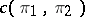of the space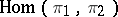of intertwining operators (cf. Intertwining operator) for two mappingsand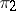of a setinto topological vector spacesand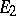, respectively. The concept of the intertwining number is especially fruitful in the case whenis a group or an algebra and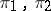are representations of. Even for finite-dimensional representations,in general, but for finite-dimensional representations,,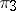the following relations hold: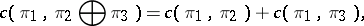while ifis a group, then alsoIf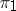andare irreducible and finite dimensional or unitary, thenis equal to 1 or 0, depending on whetherandare equivalent or not. For continuous finite-dimensional representations of a compact group, the intertwining number can be expressed in terms of the characters of the representations (cf. also Character of a representation of a group).

How to Cite This Entry:
Intertwining number. A.I. Shtern (originator), Encyclopedia of Mathematics. URL: http://encyclopediaofmath.org/index.php?title=Intertwining_number&oldid=13682
This text originally appeared in Encyclopedia of Mathematics - ISBN 1402006098